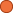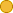## ← 01-06 Fixed Sized Queue

• 2 Followers
• 72 Lines

01-06 Fixed Sized Queue

### Get Embed Code x Embed video Use the following code to embed this video. See our usage guide for more details on embedding. Paste this in your document somewhere (closest to the closing body tag is preferable): ```<script type="text/javascript" src='https://amara.org/embedder-iframe'></script> ``` Paste this inside your HTML body, where you want to include the widget: ```<div class="amara-embed" data-url="http://www.youtube.com/watch?v=m4BhRDDG2oQ" data-team="udacity"></div> ``` 2 Languages

•English [en] original | incomplete
•Arabic [ar] incomplete

Showing Revision 2 created 07/16/2014 by Udacity Robot.

1. What I'm going to do now is go over a fixed-sized queue data structure
2. that's going to serve as a running example for some of this lecture,
3. and we're also going to keep using for a couple of our exercises for the next several units.
4. And the way this data structure works is it supports an enqueue operation,
5. a dequeue operation, an enqueue elements to dequeue and FIFO order.
6. The FIFO is first in first out--so if we enqueue 7 and then also 8, the first thing that we dequeue.
7. will be 7 then 8, and if we try to dequeue again--if we try do dequeue an element
8. with an empty queue, we're going to get some sort of an error.
9. Okay, so that's all fixed-sized queue. Now let's look at some code.
10. So in the implementation site--what we have here is a Python object so it's called queue
11. and the constructor for that object is going to set up the data structure because structure
12. for that object is going to take a size max argument--that's going to determine the largest
13. number of objects that can be stored in our queue and it's going to set up the data structure.
14. So first it's going to make sure that size max is greater than zero.
15. It's going to save a temporary copy of that, initialize some head and tail pointers,
16. a size variable which stores the number of objects currently in the queue,
17. and finally we need to reserve some space for the queque elements themselves.
18. But you can see here is we're allocating a Python array and so one implementation option
19. for a queue in Python will be to just use a Python list and that will be basically trivial.
20. Python list already is pretty much need of a support enqueue and dequeue operations
21. and the problem with the Python list is they're dynamically allocated,
22. they are dynamically tight and that makes them not very fast.
23. And so by allocating a fixed-sized storage region of statically tight memory,
24. where the "i" here means that our queue is only going to be able to store integers
25. we can avoid some of Python's dynamic checks that makes the queue slow
26. and so in some cases, a queue based on a Python list is perfectly fast but on the other hand
27. in some benchmarking that I did, this statically sized, statically tight queue
28. is more than 10 times faster than a queue based on a Python list.
29. So the first method that queue supports is the empty method and this simply returns true if
30. self.size equals zero--so of course the empty queue is the one that currently contains zero elements.
31. Very similarly, the full queue method returns true if the current size of the queue
32. is equal to the maximum size of the queue.
33. So now let's look at a couple of routines that are slightly trickier.
34. The enqueue method is going to take an argument x.
35. X is an integer that we want to try add to the queue.
36. The first thing this method is going to do is check if the current size of the queue
37. is the maximum size--if so the queue is full, then we're going to return false.
38. If we passed this test, of course, the queue is not full and we have room.
39. So the next thing we're going to do is put the argument data item
40. into the queue at the location pointed to by the tail.
41. And so now let me show you a little bit of about how our representation works.
42. So for demonstration purposes, we're going to look at a 3-element queue
43. and initially it's going to have a head and a tail according to the first queue element--
44. that is the queue element with index zero and also its size is going to be zero.
45. To enqueue an item, the first check will be useful--no it's not because its size is zero.
46. We go ahead and put the item--let's say it's the number 7 in the queue element pointed to by the tail
47. We're never going to increment the tail, and now the last thing we have to do to enqueue
48. an element is increase the size of the queue to be 1.
49. Okay, now let's go look back at the code.
50. Seeing here at the code, we can see that we put the element in the queue,
51. we increased the size of the queue, we moved the tail to point to the next element
52. and the only thing that's left the only bit of logic that's sort of tricky here is
53. if the tail of the queue point passed the end of the queue--that is to say if it's equal to the max
54. and so remember what the zero index array, the maximum way in is going to be one pass
55. at the end of the queue--we're going to reset the tail to point at the zero element of the queue--
56. that is to stay at the beginning.
57. Now the dequeue operation is very similar--first if the size of the queue is zero then the queue
58. is empty, we're not going to be able to dequeue an item.
59. And so what we're going to do in this case is return Python to none type.
60. So none of the special data types supported by Python we can often use to indicate
61. that we don't have anything--we don't have any actual value.
62. So if we pass that test, then there is something to return.
63. So what we're going to do is store the item from the head of the queue in a temporary variable.
64. So x is going to get 7. We're going to decrement the size of the queue.
65. We're going to move the tail point out to point the next element and then using logic very similar
66. to the tail pointer in the enqueue function, we're going to wrap the head pointer around
67. if it's gone passed the end of the queue.
68. So let's go back to the drawing and look out and see how this plays out.
69. So we're going to return 7, decrement the size, and make the head element
70. point to the next element of the list, and we're not going to bother erasing the 7 we returned
71. but we're going to have to make sure that our queue logic never returns this dead element.
72. Oh! So let's take a very quick quiz just to make sure that you understood all of that.